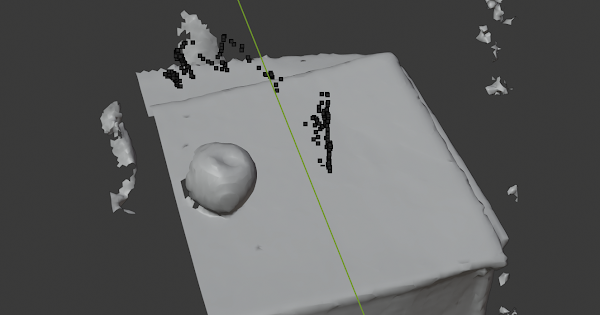# depthFrame x,y,z to world coordinates and model coordinates

I have tried searching the forum and tried digging through samples to figure out how get world coordinates and the model coordinates from a depthFrame point (x,y,z). I have been trying to take a depthFrame (x,y,z) (x in pixels, y in pixels, and z in millimeters) and then using the STTracker lastCameraPose, glProjectionMatrix, iOSColorFromDepthExtrinsics. I feel like there should be enough here to figure it out, but I am confused on how to do this. Does anyone have a solid example of how to do this?

Here is an image of what I am trying to do…https://photos.app.goo.gl/9intVNP8TUSKeADz9. I want to find an object using ML, then identify where it is in the world, and the model that is being created.

This work from @9gel was from a while ago, maybe a starting point for what you want to do?

Thanks a bunch for the tip!

I am able to find an object in the IOS color frame and using the SLAM tracking and depth, find the point in 3D world space. I need to convert that to the model space that gets written to the .obj file. It looks like I have to do some scaling, rotating and translation. But I have no idea what matrix to use.

Here is my code to find points in World Space

func getXYZInWorldSpaceFromPixels(pixelX: Float, pixelY: Float, intrinsics: STIntrinsics, depthInMeters: Float, cameraPose: GLKMatrix4) -> GLKVector3 {

// these come from camera intrinsics which is on STDepthFrame.intrinsics

let fx = intrinsics.fx

let fy = intrinsics.fy

let cx = intrinsics.cx

let cy = intrinsics.cy

// might have to do something with skew…the values I got from the intrinsics in this example were 0 so I didn’t do anything with skew

let X = depthInMeters * (pixelX - cx) / fx

let Y = depthInMeters * (cy - pixelY) / fy

let Z = depthInMeters

let worldSpacePoint = GLKMatrix4MultiplyVector3WithTranslation(cameraPose, GLKVector3Make(X, Y, Z))

return worldSpacePoint

}

Here are my examples…

the black dots are the points I have found and they are the world space coordinates.I have manually taken the black dots above and scaled, rotated and translated them to fit where they should be in the model space.

Here are my STMapper options:

let mapperOptions: [String: Any ] = [

kSTMapperVolumeResolutionKey: NSNumber(floatLiteral: 0.005),

kSTMapperVolumeBoundsKey: NSArray(array: [600,600,600])

]

It appears that I need to scale my points by 1/3, which could make sense from my options if it is to fit within a 1X1X1 cube which is an assumption I am making. But then I am not sure what I need to do to translate it…

I assume this is all known by the Structure team since this is being done (taking world depth and converting to model space in this .obj file)

Please and with great thanks help me figure this out!